## Conservation Of Momentum

CONSERVATION OF MOMENTUM

As I said before to give something acceleration we must apply an external force. If there is no force then object continue its motion. For momentum change we must apply impulse, in other words there must be external applied force to change momentum of the object. If there is no force applied then momentum of the system is conserved in magnitude and direction.

Pinitial=Pfinal

To understand conservation of momentum we will examine a collision of two objects. Look at the given picture, two ball having masses m1 and m2 and velocities V1 and V2 collide. If there is no external force acting on the system; momentum of the system is conserved.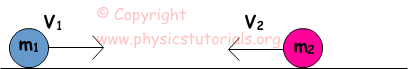During the collision balls exert force to each other. From the Newton’s third law these forces are equal in magnitude and opposite in direction. We can say action and reaction to these forces. Picture given below shows these forces at the instance of collision.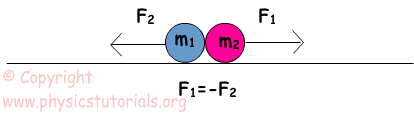Ball having m2 mass exerts force on m1 and ball having m2 mass exerts force on m1. Since collision occurs from the interaction of the bodies then time of collision or interaction must be equal.

F1=-F2 and t1=t2

Impulses of the balls are;

Impulse1=F1. t1 and Impulse2= -F2. t2

F1. t1 = -F2. t2

Impulses of the balls are equal in magnitude. As we said before impulse is equal to the change of momentum. Thus, we can say that momentum changes of the balls are also equal in magnitude and opposite in direction.

m1 (V1final-V1initial) =-m2 (V2final-V2initial)

Conservation of momentum law says that one object loses momentum and other one gains it. Total momentum of the system is conserved.

Example: Bullet shown in the picture collides to a fixed block.0, 2 s is the interaction time of bullet with block. If the velocity of the bullet is 250m/s after the collision, find the resistance of the block to the bullet.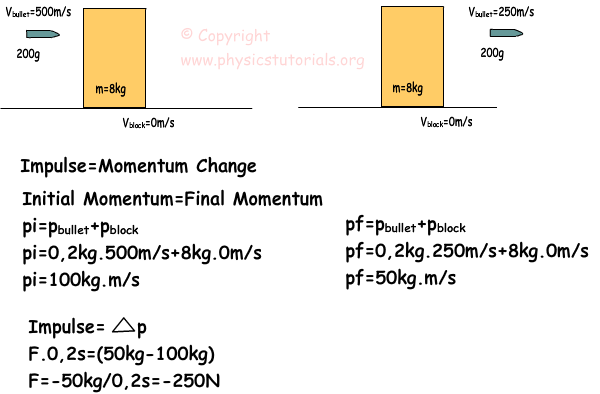Resistance of the block to the bullet is -250N. We use conservation of momentum to find the change in momentum and using the impulse momentum equation we find force that block apply to bullet.

Example: Two cars are stationary at the beginning. If the car having 10kg mass starts to move to the east with a velocity of 5m/s, find thevelocity of the car having mass 4kg with respect to the ground.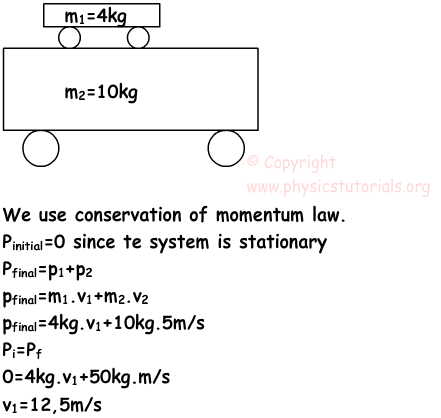V1 is 12,5m/s to the west. If you want to find the velocity of the small car with respect to big car you should benefit from relative motion and do your calculations according to the vector properties, in other words you must consider the directions of the velocities.

Example: Two blocks move with the given velocities. When they collide they stick and move together.Find the velocity of the blocks after the collision.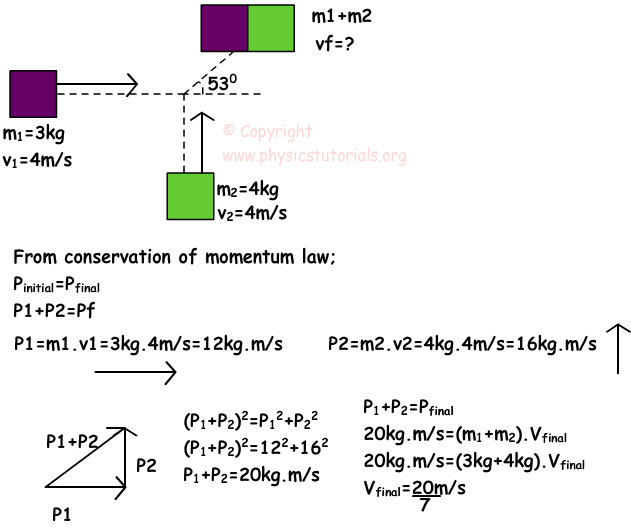Example: Two bullets having velocities 550m/s and 200m/s moves towards to a block that having mass 12kg. If the bullets stick to the block and they move together find the velocity of the final system.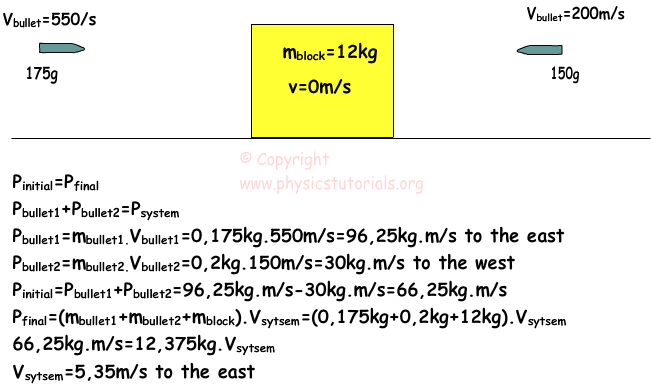We find momentum of the bullets first then; we calculate total initial momentum by adding them using their vector directions. Finally using conservation of momentum law we find the velocity of the system.

Author:

Tags: